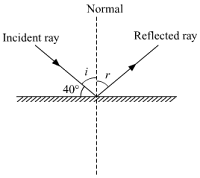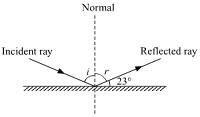☰ अधिक माहितीसाठी येथे क्लीक करा

Question 1: Fill in the blanks i. The perpendicular to the mirror at the point of incidence is called....................... . ii. The reflection of light from a wooden surface is.................. reflection. iii. The working of Kaleidoscope is based on the properties of................... . Answer 1: i. The perpendicular to the mirror at the point of incidence is callled normal. ii. The reflection of light from a wooden surface is diffused reflection. iii. The working of Kaleidoscope is based on the properties of reflection of light. Question 2: Draw a figure describing the following. The reflecting surface of two mirrors make an angle of 90∘ with each other. If a ray incident of one mirror has an angle of incidents of 30∘, draw the ray reflected from the second mirror. What will be its angle of reflection? Answer 2: Let r2 be the angle of reflection from the second mirror.From laws of reflection, ∠i1=∠r1=30o∠i1=∠r1=30o Now, in ΔABC∆ABC , θ=180o−90o−60o=30o⇒i2=60oHence,r2=60oθ=180o-90o-60o=30o⇒i2=60oHence, r2=60o The angle of reflection of incident ray is 60∘. Question 3: How will you explain the statement 'we cannot see the objects in a dark room? Answer 3: Light enables us to see objects around us. We see an object when light falls on it and gets reflected from its surface and enters our eyes. In a dark room, there is no source of light. Thus, no light falls on the surface of objects and hence we do not see them. This is why we cannot see the objects in a dark room. Question 4: Explain the differences between regular and irregular reflection light. Answer 4: Regular reflection of light Irregular reflection of light This type of reflection occurs on smooth surfaces such as a plane mirror. This type of reflection occurs on rough surfaces such as a black board. In this type of reflection, the reflected rays are parallel to each other. In this type of reflection, the reflected rays are non-parallel to each other. In this type of reflection, the reflected rays are in one direction. In this type of reflection, the reflected rays are in different direction. Question 5: Draw a figure showing the following a. Incident Ray b. Angle of incidence c. Angle of reflection d. Point of incidence e. Reflected ray Answer 5:Question 6: Study the following incident. Swara and Yash were looking in a water filled vessel. They could see their images clearly in the still water. At that instant, Yash threw a stone in the water. Now their images were blurred. Swara could not understand the reason for the blurring of the images. Explain the reason for blurring of the images to swara by answering the following questions. i. Is there a relation between the reflection of light and the blurring of the images.? ii. Which types of reflection of light can you notice from this? iii. Are laws of reflection followed in these types of reflection? Answer 6: i. Yes, there is a relation between the reflection of light and blurring of the images. ii. Initially, the water was still and its surface was smooth. Thus, regular type of reflection was taking place from its surface i.e. all the light rays coming from Swara's body or Yash's body reflected in the same direction on hitting the water surface and reached our eyes. Because of this, we saw a clear image. But when Yash threw a stone in the water, the water surface became rough due to which irregular type of reflection of light occurred on its surface .i.e all the light rays coming from Swara's body or Yash's body reflected in different directions on hitting the water surface. Thus, only some part of the reflected rays reached our eyes. Because of this, we see a blurred image. iii. Yes, laws of reflection are followed in both the types of reflection. Question 7: Solve the following examples. a. If the angle between the plane mirror and the incident ray is 40∘, what are the angles of incidence and reflection? b. If the angle between the mirror and reflected ray is 23∘, what is the angle of incidence of the incidence ray? Answer 7:a. ∠i + 40o=90o⇒∠i=50oUsing law of reflection, we have∠i=∠r=50o∠i + 40o=90o⇒∠i=50oUsing law of reflection, we have∠i=∠r=50o b.∠r+ 23o=90o⇒∠r=67oUsing law of reflection, we have∠i=∠r=67o

STD 8 SCIENCE 16. Reflection of LightReviewed by Amol Uge on February 19, 2019 Rating: 5